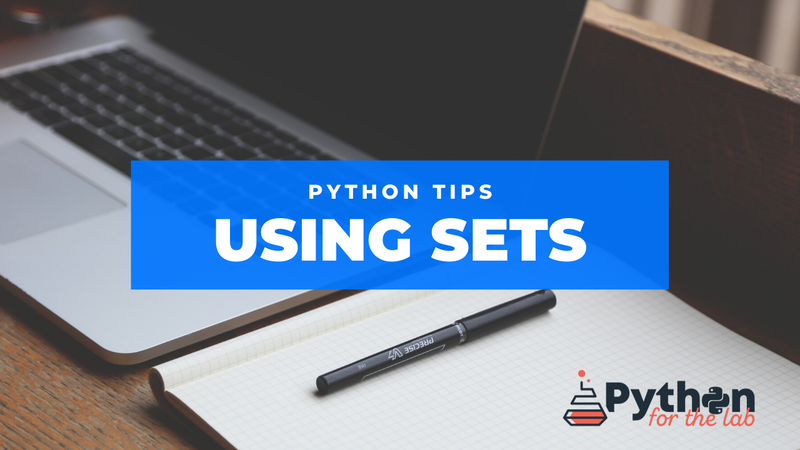#### Get all the information directly to your inbox

Get relevant information, unsubscribe at any time.# Python Tips: Using Sets

### Go beyond lists and dictionaries, learn how to use sets

May 11, 2020 tips data-types sets

Most people are familiar with lists, tuples, and dictionaries as the basic data types for grouping information. However, there is another convenient option: sets. They are directly linked to the mathematical idea of a set. To define them, we can use the following syntax:

``var = {1, 2, 3, 4}``

We can also use this alternative:

``var1 = set([1, 2, 3, 4])``

Where the argument of `set` is an iterable (see this article if you want to learn about iterables).

Sets can't have repeated elements. Therefore, they can be used to clean up the repeated elements from iterables. For example,

``````mis = "mississippi"

var = set(mis)
for i in var:
print(i)
# p
# i
# m
# s``````

The code only outputs different letters. See that the order is not respected. By definition, sets are unordered. Sets also define operations.

### Operations Between Sets

#### Union

To calculate the union between two sets:

``````mis = "mississippi"
ama = "amazon"
var1 = set(mis)
var2 = set(ama)

letters = var1 | var2

for i in letters:
print(i)

# n
# i
# s
# a
# m
# z
# p
# o``````

#### Intersection

The intersection between two sets can also be calculated with an operator:

``````mis = "mississippi"
ama = "amazon"
var1 = set(mis)
var2 = set(ama)

letters = var1 & var2

for i in letters:
print(i)

# m``````

#### Subsets or Supersets

If you want to know whether a set is contained in another (or the other way around), you can use the comparison operator:

``````var1 = set('asia')
var2 = set('australia')

print(var1 < var2)

# True``````

#### Difference between sets

The difference between sets can be defined in different ways. For example, if we want to remove the elements of one set from another, we can do the following:

``````var1 = set('america')
var2 = set('australia')

var3 = var2 - var1

for i in var3:
print(i)

# t
# s
# l
# u``````

It removed the elements of `var1` from `var2`. There is no need for `var1` to be a subset of `var2`, it just removes the elements that are present and disregards the ones that are not.

But there is another option, which is the symmetric difference, meaning the elements that are present in only one of the two sets, but not in both:

``````var1 = set('america')
var2 = set('australia')

var3 = var1 ^ var2

print(var3)

# {'c', 'u', 's', 'm', 'e', 't', 'l'}``````

### Accessing Elements

Since sets are unordered, we can't access the elements using an index. However, sets are iterables, which allowed us to do things like

``````for i in var1:
print(i)``````

Sets also allow us to pop elements:

``````var = set('mississippi')

print(var)
print(var.pop())
print(var)

# {'s', 'i', 'm', 'p'}
# s
# {'i', 'm', 'p'}``````

We can also verify if an element is contained within a set:

``````var = set('mississippi')
print('i' in var)

# True``````

### Frozen Sets

Sets are mutable, which means that we can change their contents while the variable will be pointing to the same object. For example:

``````var1 = set('mississipi')
var2 = var1
var3 = set('amazon')

print(var2)
# {'s', 'p', 'i', 'm'}
var1 |= var3

print(var2)
# {'p', 'i', 'n', 'm', 'o', 'z', 's', 'a'}``````

In the example above, we change `var1` but the changes get propagated to `var2`, as expected for mutable objects. Sets define another data type that prevents that behavior, called `frozenset`, see the example below:

``````var1 = frozenset('mississipi')
var2 = var1
var3 = set('amazon')

print(var2)
# frozenset({'p', 'm', 's', 'i'})
var1 |= var3

print(var2)
# frozenset({'p', 'm', 's', 'i'})``````

We can also check the `id` of the variables to see that they are effectively changing:

``````var1 = frozenset('mississipi')
print(id(var1))
# 139836399804936
var1 |= var3
print(id(var1))
# 139836371992136``````

### Support Us

If you like the content of this website, consider buying a copy of the book Python For The Lab